# 机器学习 —— 模型和代价函数

Machine Learning —— Model & Cost Function

## 模型表示 Model Representation——简介

• Notation
m –训练样本的个数
x – 输入数据/特征
y – 输出数据/目标数据

• 训练数据
$\left(x,y\right)$$(x, y)$
或者表示为：
$\left({x}^{\left(i\right)},{y}^{\left(i\right)}\right)$$(x^{(i)}, y^{(i)})$ $i$$i$表示第$i$$i$个训练样本

• 训练过程：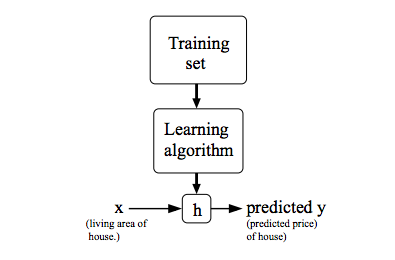Training Set -> Learning Algorithm ->h函数(hypothesis, 该假设函数的确定来源于x和y之间的映射关系)

• h的表示形式：
假设为一个变量的线性回归的情况下
${h}_{\theta }\left(x\right)={\theta }_{0}+{\theta }_{1}x$$h_{\theta}(x)={\theta}_0+{\theta}_1x$

## 代价函数 Cost Function——定义

（题外话）代价函数 cost function, 损失函数 loss function和目标函数 object function 的简单说明

$\mid {y}_{i}-f\left({x}_{i}\right)\mid$

$\frac{1}{N}\cdot \sum _{i=1}^{N}\mid {y}_{i}-f\left({x}_{i}\right)\mid$

$\frac{1}{N}\cdot \sum _{i=1}^{N}\mid {y}_{i}-f\left({x}_{i}\right)\mid +正则化项$

$mi{n}_{{\theta }_{0},{\theta }_{1}}\frac{1}{2m}\sum _{i=1}^{m}\left({h}_{\theta }\left({x}^{\left(i\right)}\right)-{y}^{\left(i\right)}{\right)}^{2}$

Cost Function:

$J\left({\theta }_{0},{\theta }_{1}\right)=\frac{1}{2m}\sum _{i=1}^{m}\left({h}_{\theta }\left({x}^{\left(i\right)}\right)-{y}^{\left(i\right)}{\right)}^{2}$

## 代价函数——Cost Function I

### Review

Hypothesis：

${h}_{\theta }\left(x\right)={\theta }_{0}+{\theta }_{1}x$

Parameters:

Cost Function:

$J\left({\theta }_{0},{\theta }_{1}\right)=\frac{1}{2m}\sum _{i=1}^{m}\left({h}_{\theta }\left({x}^{\left(i\right)}\right)-{y}^{\left(i\right)}{\right)}^{2}$

Goal: $minimiz{e}_{{\theta }_{0},{\theta }_{1}}J\left({\theta }_{0},{\theta }_{1}\right)$$minimize_{\theta_0, \theta_1}J(\theta_0,\theta_1)$

### Example

(用简单的假设函数了解代价函数的工作原理)

Hypothesis：

${h}_{\theta }\left(x\right)={\theta }_{1}x$

Parameters:

${\theta }_{1}$

Cost Function:

$J\left({\theta }_{1}\right)=\frac{1}{2m}\sum _{i=1}^{m}\left({h}_{\theta }\left({x}^{\left(i\right)}\right)-{y}^{\left(i\right)}{\right)}^{2}$

Goal: $minimiz{e}_{{\theta }_{1}}J\left({\theta }_{1}\right)$$minimize_{\theta_1}J(\theta_1)$

### 真实图例：

• ${\theta }_{1}=0$$\theta_1=0$的情况下假设函数和代价函数图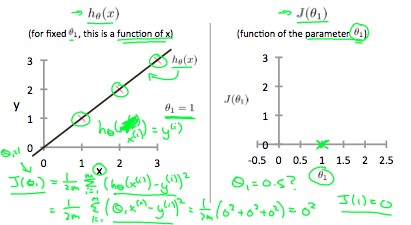• ${\theta }_{1}=0.5$$\theta_1=0.5$的情况下假设函数和代价函数图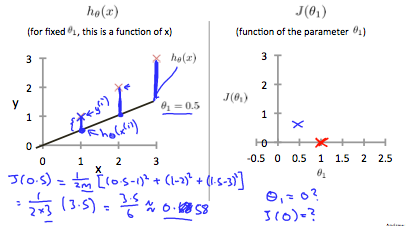• 所有参数和代价函数的对应图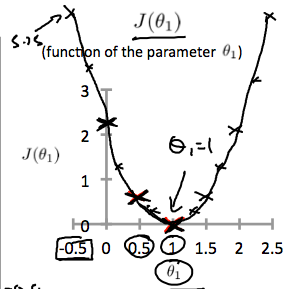根据以上图例，我们得知，要取得代价函数的最小值，应该选择${\theta }_{1}=1$$\theta_1=1$的参数表示。

## 代价函数——Cost Function II

### Example

(有两个参数情况下的假设函数以及代价函数)

Hypothesis：

${h}_{\theta }\left(x\right)={\theta }_{0}+{\theta }_{1}x$

Parameters:

Cost Function:

$J\left({\theta }_{0},{\theta }_{1}\right)=\frac{1}{2m}\sum _{i=1}^{m}\left({h}_{\theta }\left({x}^{\left(i\right)}\right)-{y}^{\left(i\right)}{\right)}^{2}$

Goal: $minimiz{e}_{{\theta }_{0},{\theta }_{1}}J\left({\theta }_{0},{\theta }_{1}\right)$$minimize_{\theta_0, \theta_1}J(\theta_0,\theta_1)$

### 真实图例

• 随机参数 $\theta$$\theta$ 对应的图例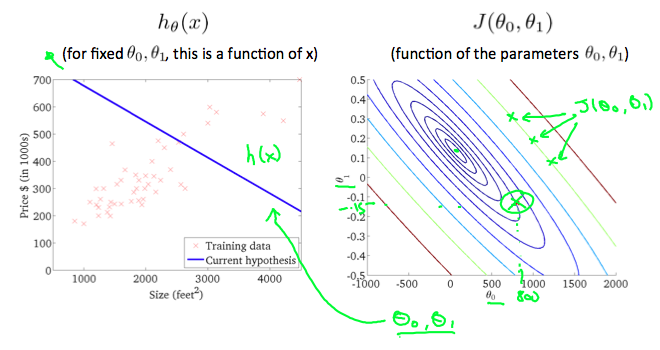• ${\theta }_{0}=360,{\theta }_{1}=0$$\theta_0=360, \theta_1=0$对应图例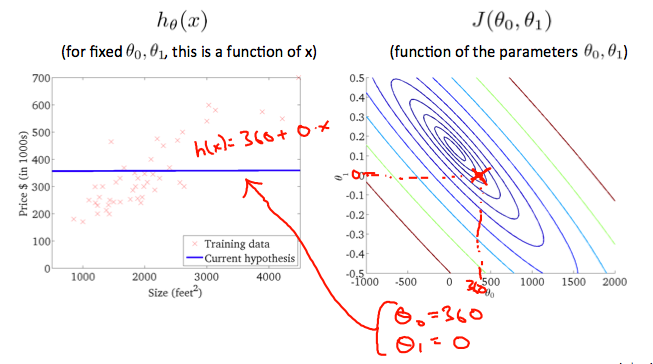• 相对接近代价函数最小值对应的图例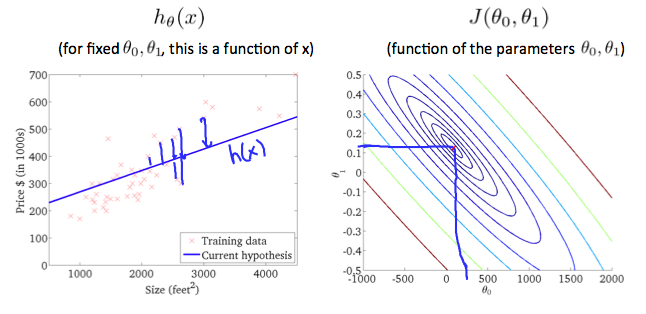Goal：找到一种算法，能够自动找到代价函数的最小值对应的参数。